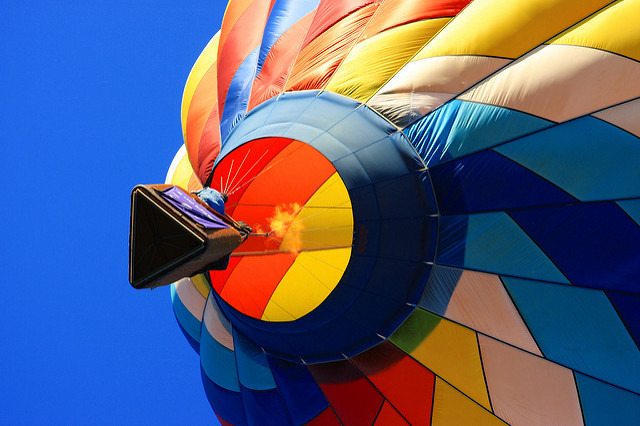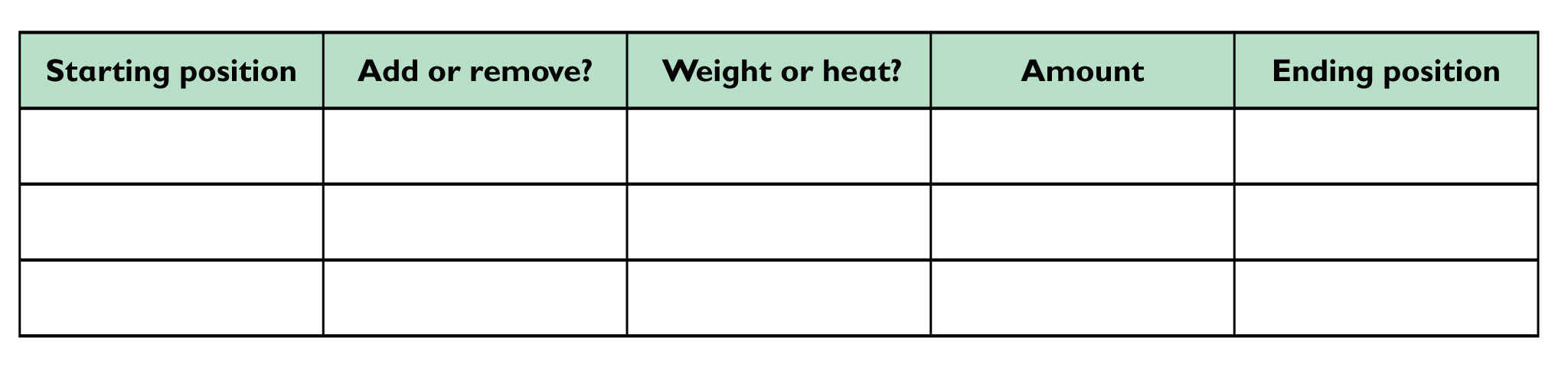Subject:
Numbers and Operations
Material Type:
Lesson Plan
Level:
Middle School
7
Provider:
Pearson
Tags:
Language:
English
Media Formats:
Text/HTML

# The Effects of Positive & Negative Numbers## Overview

Students explore what happens to a hot air balloon when they add or remove units of weight or heat. This activity is an informal exploration of addition and subtraction with positive and negative integers.

# Key Concepts

This lesson introduces a balloon simulation for adding and subtracting integers. Positive integers are represented by adding units of heat to air and negative integers are represented by adding units of weight. The balloon is pictured next to a vertical number line. The balloon rises one unit for each unit of heat added or each unit of weight removed. The balloon falls one unit for each unit of weight added or each unit of heat removed from the air.

Mathematically, adding 1 to a number and subtracting −1 from a number are equivalent and increase the number by 1. Adding −1 to a number and subtracting 1 from a number are equivalent and decrease the number by 1. Addition and subtraction with positive and negative numbers are explored formally in the next several lessons.

# Goals and Learning Objectives

• Explore the effects of adding or subtracting positive and negative numbers.

# Lesson Guide

Demonstrate the Hot Air Balloon interactive. Show students how to add or remove weight or heat to move the balloon. Give students a minute or two to experiment on their own and then discuss the results of their experimentation with a partner.

# Hot Air Balloon Simulation

Have you ever wondered how a hot air balloon works?

The process is actually pretty simple. A hot air balloon has a propane burner that heats the air in the balloon. Because hot air is lighter than cold air, the balloon rises. When hot air cools inside the balloon, the balloon goes down.

Weight also affects the rising and falling of a hot air balloon. If weight is removed, the balloon rises.

The Hot Air Balloon interactive is a basic simulation of how a hot air balloon works. Remember a simulation models the situation but every aspect does not have to be real—in this case, you could not add weight in midair.

Experiment with the simulation by performing the indicated actions. Discuss your observations with your partner.

In this simulation, the hot air balloon begins high in the air. We call this position 0 because it is the hot air balloon's starting position.

• Start at 0. Add 4 units of heat to the air.
• Start at 0. Add 5 units of weight.
• Start at 0. Remove 8 units of heat from the air.
• Start at 0. Remove 4 units of weight

INTERACTIVE: Hot Air Balloon

# Lesson Guide

Discuss the Math Mission. Students will explore how adding or removing heat and weight affects the altitude of a hot air balloon.

## Opening

Explore how adding or removing heat and weight affects the altitude of a hot air balloon.

# Lesson Guide

Have students work in pairs. You might suggest that they set up a table to record data.

ELL: Partner work provides students with opportunities to negotiate meaning, share ideas, and communicate with others during problem solving. This type of language-rich environment encourages students to work together and learn from one another, which is essential for ELLs’ learning.

SWD: Students with disabilities may have difficulty interpreting the visual information. Provide students with tactile 3-D manipulatives.

# Interventions

Student has difficulty making predictions.

• Try adding or removing weight. Will the balloon move up or down when you do this? How many units will the balloon move? If you count that many units up [or down] from the last position, where do you end up?
• Try adding or removing heat. Will the balloon move up or down when you do this? How many units will the balloon move? If you count that many units up [or down] from the last position, where do you end up?

• Students may want to set up a table to organize their work. Sample table:• Answers will vary. Students will record their results.
• Answers will vary. Students will record their results.
• Possible statement: Adding x units of heat or removing x units of weight will cause the hot air balloon to rise x units. Removing x units of heat or adding x units of weight will cause the hot air balloon to sink x units.

# Explore the Hot Air Balloon Simulation

Work with a partner on the Hot Air Balloon activity. Record your results.

• Start at 0. Add any amount of one quantity (heat or weight). Then add any amount of the other quantity. Repeat these steps several times. Sometimes keep the numbers you use the same and sometimes make them different.
• Start at 0. Add any amount of one quantity (heat or weight). Then remove any amount of the other quantity.
• Start at 0. Remove any amount of one quantity (heat or weight). Then add any amount of the other quantity.
• Continue to experiment with adding and removing quantities until you can predict what will happen if you add/remove any amount of heat or any amount of weight. Write a general statement that describes your predictions.

INTERACTIVE: Hot Air Balloon

## Hint:

Try to visualize the movements of the balloon. Which actions cause the balloon to move up? Which actions cause it to move down?

# Lesson Guide

Students should work continue to work in pairs.

# Interventions

Student has difficulty getting to zero.

• How many units would you have to move up to zero?
• What would you need to add or subtract to get to zero?
• Do you need to add or remove weight or heat? Which would move it up?

• Answers will vary. Possible responses:
• Add 5 units of heat.
• Remove 5 units of weight.
• Add 3 units of heat, then remove 2 units of weight.
• Remove 3 units of weight, then add 2 units of heat.

# From Negative 5 to 0

Suppose the balloon is at −5.

• Describe two different ways that you could get the balloon to 0 by adding or removing weight or heat.

INTERACTIVE: Hot Air Balloon

## Hint:

• In what direction do you need to move the balloon?
• How can you do this by adding or removing heat?
• How can you do this by adding or removing weight?

# Lesson Guide

Students should continue to work in pairs.

# Interventions

Student has difficulty getting to zero.

• How many units would you have to move down to zero?
• What would you need to add or subtract to get to zero?
• Do you need to add or remove weight or air? Which would move it down?

• Add 7 units of weight.
• Remove 7 units of heat.
• Add 3 units of weight, then remove 4 units of heat.
• Remove 3 units of heat, then add 4 units of weight.

# From Positive 7 to 0

Suppose the balloon is at 7.

• Describe two different ways that you could get the balloon to 0 by adding or removing weight or heat.
• In what direction do you need to move the balloon?
• How can you do this by adding or removing heat?
• How can you do this by adding or removing weight?

INTERACTIVE: Hot Air Balloon

## Hint:

You need to move the balloon down 7 units. How can you do this by adding or removing heat? How can you do this by adding or removing weight?

# Prepare for Ways of Thinking

Look for students who understand and can explain that adding weight and subtracting heat give the same result. Have these students present during Ways of Thinking.

SWD: As students present their solutions, make connections between different solutions to the same problem. This allows students to see the multiple ways to solve or analyze a problem. Write down all of the important connections, and have students copy them into their Notebook.

# Prepare a Presentation

Summarize what you learned about how adding or removing units of heat and weight affect the movement of a hot air balloon.

• Be prepared to explain and justify your predictions about what will happen to the balloon if you add or remove any amount of heat or weight.

# Lesson Guide

Select a few groups to present. Make the explicit connection between positive and negative numbers to heat and weight, if the presentations do not include this. You might ask a few specific questions about the balloon.

Possible questions:

• If the balloon is at –3 and we add 3 units of heat, where will the balloon end up?
• If the balloon is at 7and I want to move it to 3, what can I do? Is there something different I can do that will have the same result?
• If the balloon is at –5 and I want to move it to 5, what can I do? Is there something different I can do that will have the same result?

# Ways of Thinking: Make Connections

## Hint:

• If your prediction is not correct, what do you think you need to change?
• If the balloon starts at a negative number, what do you have to do with regard to heat in order to get the balloon to 0? Why?
• If the balloon starts at a positive number, what do you have to do with regard to weight in order to get the balloon to 0? Why?